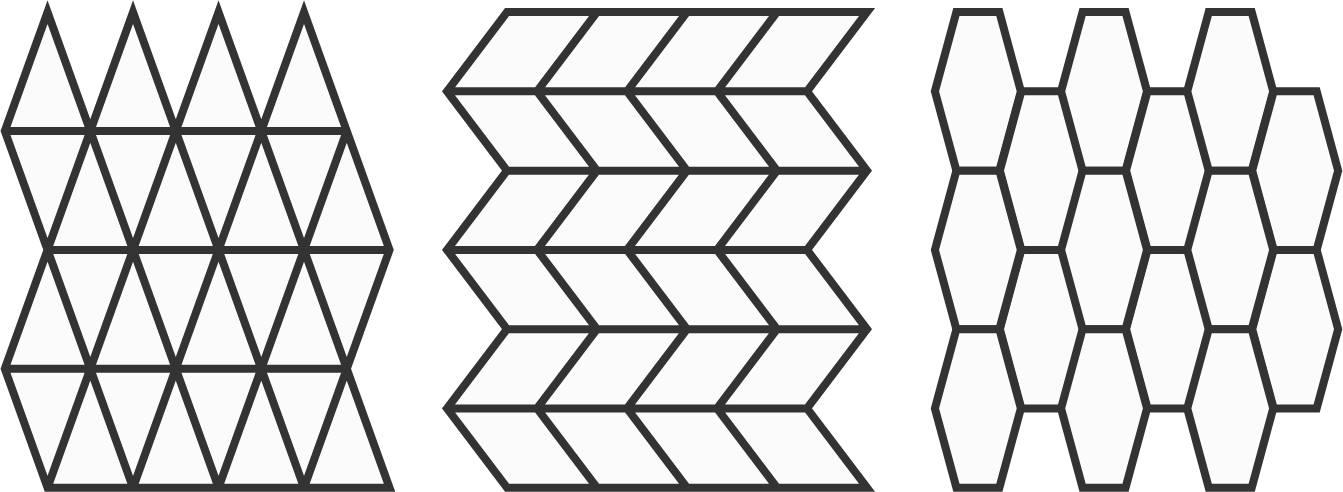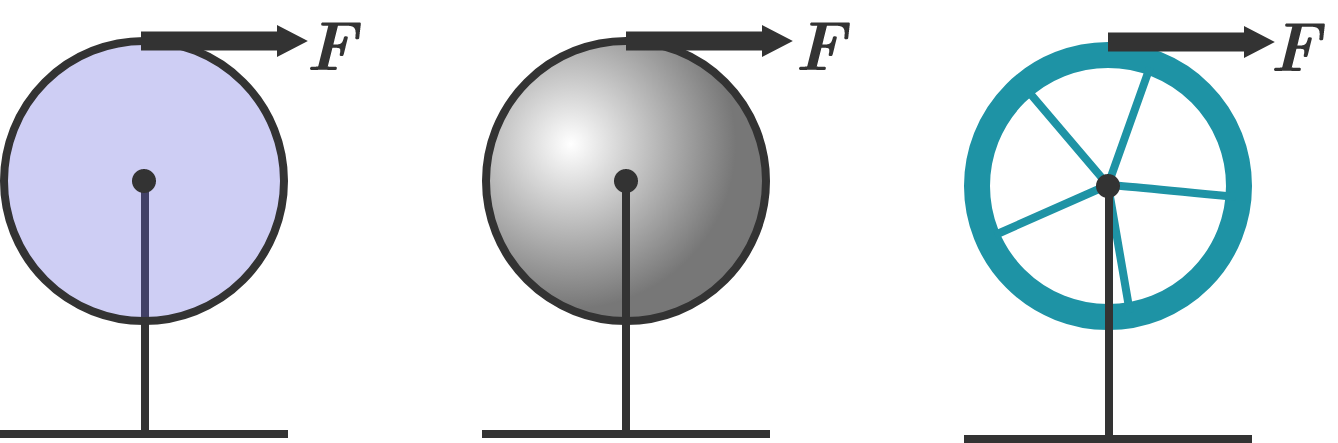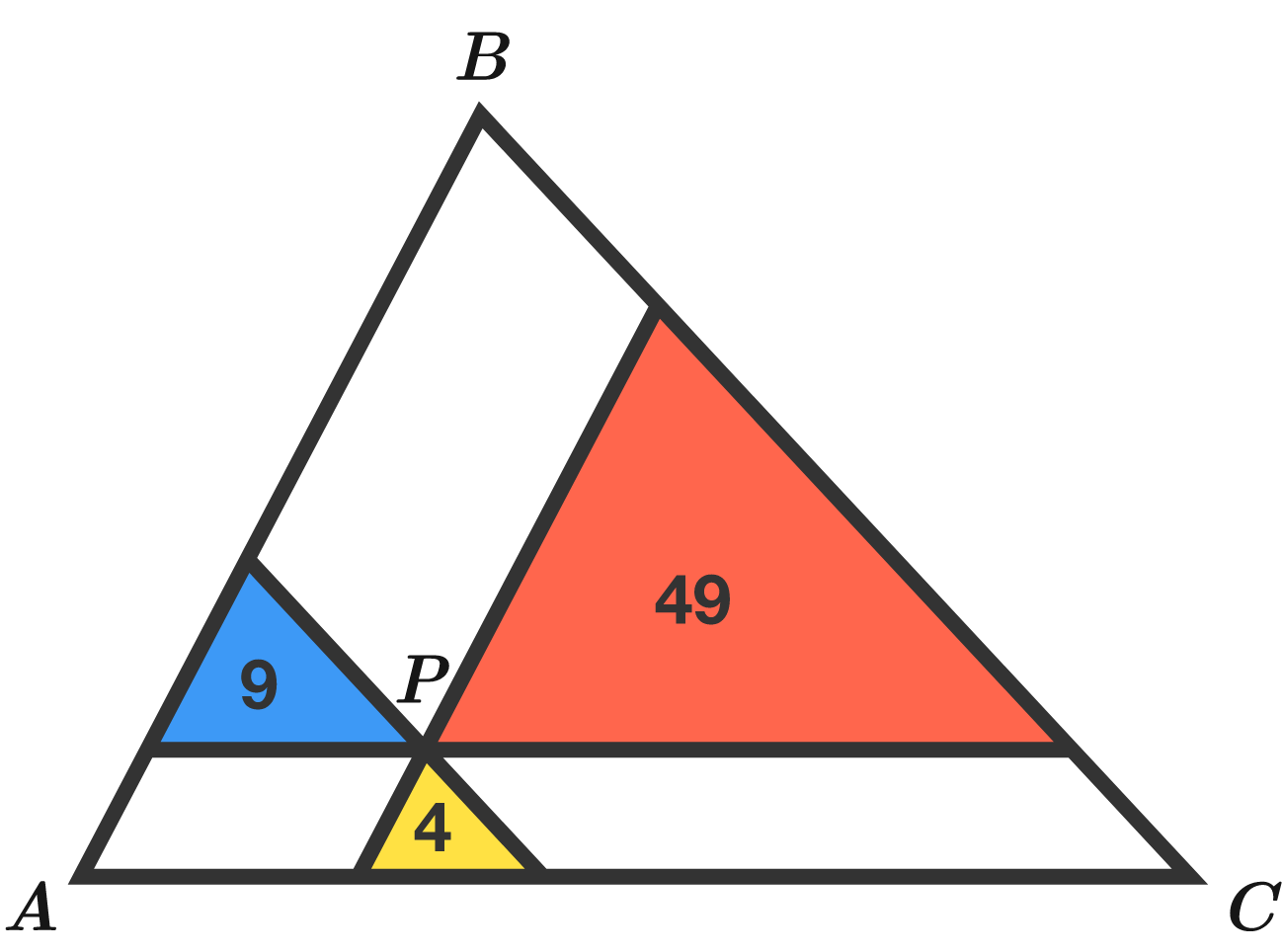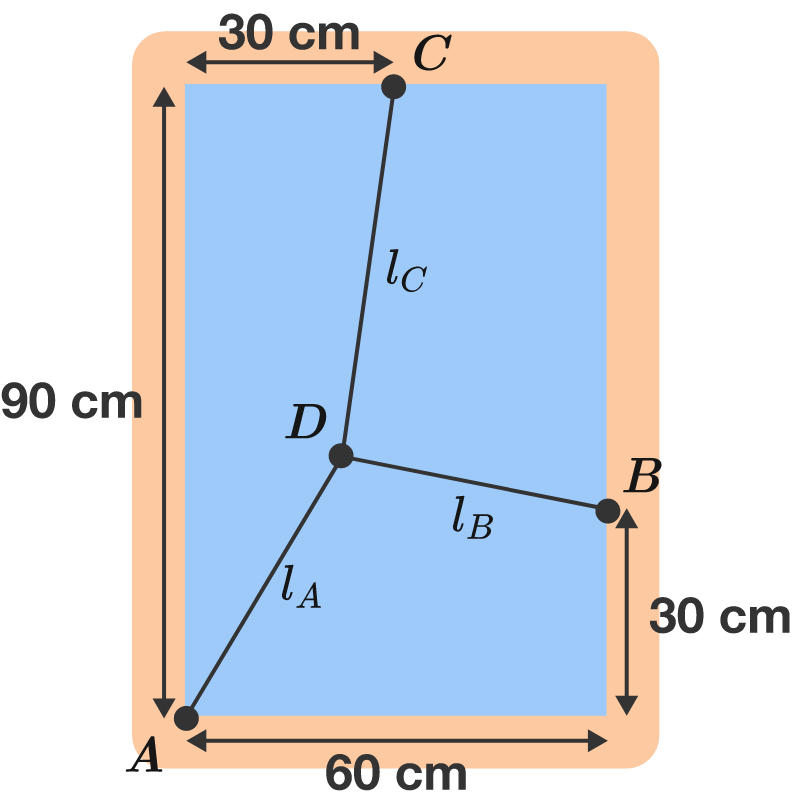# Problems of the Week

Contribute a problem

# 2018-06-18 Intermediate

An infinite plane can be tiled using identical triangles, quadrilaterals, or hexagons with no overlaps and no gaps.Is it possible to tile an infinite plane using identical pentagons?

A solid disk, a solid sphere, and a hoop of identical mass $m$ and radius $R$ are mounted at rest on frictionless axles. Each object has a string wrapped around its circumference, and the strings are each pulled with an identical force $F$ for the same time interval; the strings do not slip.

Which object will have the greatest rotational kinetic energy at the end of the time interval?Note: The mass of the spokes on the hoop is negligible.

Lines are drawn through point $P$ parallel to the sides of triangle $ABC.$ The resulting 3 triangles have areas 4, 9, and 49.

What is the area of triangle $ABC?$The areas of these 3 smaller triangles are 4, 9, and 49. What's the area of the big, outer triangle?In this rectangular window frame, a spider begins to weave its web. The basic framework consists of three threads, which start from points $A, B,$ and $C$ and are glued together at point $D.$

What is the length $l_A$ of the first thread in centimeters?

Assumptions: Without tension, all threads have the same length, but they are stretched many times their original length. Thus, the tension force $F_i \approx - k l_i$ acting along a thread is approximately proportional to its total length $l_i$. While the lengths $l_i$ of the threads can be different, the spring constant $k$ is the same for all threads. Apart from the tension, no further forces act on the spider threads.

$a,b,$ and $x$ are real numbers satisfying the following system of equations: \begin{aligned} a+b &=& 3x-2 \\ ab &=& 4x^2-3x-4. \end{aligned} What is the minimum value of $a^2+b^2$ (to three decimal places)?

×

Problem Loading...

Note Loading...

Set Loading...